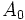# Quotient-iterated omega subgroup of group of prime power order

## Contents

BEWARE! This term is nonstandard and is being used locally within the wiki. [SHOW MORE]
This article defines a subgroup property: a property that can be evaluated to true/false given a group and a subgroup thereof, invariant under subgroup equivalence. View a complete list of subgroup properties[SHOW MORE]

## Definition

Suppose$p$ is a prime number and$G$ is a finite$p$-group, so$G$ is a group of prime power order. A subgroup$H$ of$G$ is termed a quotient-iterated omega subgroup of$G$ if there exist nonnegative integers$a_1,a_2,\dots,a_r$ and normal subgroups$A_0,A_1,A_2,\dots,A_r$ of$G$, where$A_0$ is trivial,$A_r = H$, and$A_i/A_{i-1} = \Omega^{a_i}(G/A_{i-1})$.

## Relation with other properties

### Stronger properties

property quick description proof of implication proof of strictness (reverse implication failure) intermediate notions
Omega subgroup of group of prime power order

### Weaker properties

property quick description proof of implication proof of strictness (reverse implication failure) intermediate notions
Fully invariant subgroup of group of prime power order
Intermediately fully invariant subgroup of group of prime power order